• 2021-10-26 17:20:08

//求角度 及前后左右方位
public void checkTargetDirForMe(Transform target)
{
//xuqiTest：  target.position = new Vector3(3, 0, 5);
Vector3 dir = target.position - transform.position; //位置差，方向
//方式1   点乘
//点积的计算方式为: a·b =| a |·| b | cos < a,b > 其中 | a | 和 | b | 表示向量的模 。
float dot = Vector3.Dot(transform.forward, dir.normalized);//点乘判断前后：dot >0在前，<0在后
float dot1 = Vector3.Dot(transform.right, dir.normalized);//点乘判断左右： dot1>0在右，<0在左
float angle = Mathf.Acos(Vector3.Dot(transform.forward.normalized, dir.normalized)) * Mathf.Rad2Deg;//通过点乘求出夹角

//方式2   叉乘
//叉乘满足右手准则  公式：模长|c|=|a||b|sin<a,b>
Vector3 cross = Vector3.Cross(transform.forward, dir.normalized);//叉乘判断左右：cross.y>0在左，<0在右
Vector3 cross1 = Vector3.Cross(transform.right, dir.normalized); //叉乘判断前后：cross.y>0在前，<0在后
angle = Mathf.Asin(Vector3.Distance(Vector3.zero, Vector3.Cross(transform.forward.normalized, dir.normalized))) * Mathf.Rad2Deg;

}  

项目中常会用到物体移动，追踪，判断两物体移动方向是否相同，两物体移动方向夹角，以及物体 A 朝 物体 B 顺时针方向还是逆时针方向移动。物体 A 在 物体 B 的前后左右方向。
下面通过点乘（Dot）、叉乘（Cross）， 得到上面的需求结果。

using UnityEngine;
using System.Collections;

public class VectorDotCross : MonoBehaviour {

// 关于点积
private void Dot()
{
/*
点积
点积的计算方式为:  a·b=|a|·|b|cos<a,b>  其中|a|和|b|表示向量的模，
<a,b>表示两个向量的夹角。 通过点积判断当两个向量的方向向是否相同
（大致相同即两个向量的夹角在 90 度范围内）
两个向量的 点积 大于 0 则两个向量夹角小于 90 度， 否则 两个向量的
夹角大于 90 度，
*/
// 定义两个向量 a、b
Vector3 a = new Vector3(1, 1, 1);
Vector3 b = new Vector3(1, 5, 1);

// 计算 a、b 点积结果
float result = Vector3.Dot(a, b);

// 通过向量直接获取两个向量的夹角（默认为 角度）， 此方法范围 [0 - 180]
float angle = Vector3.Angle(a, b);

// 下面获取夹角的方法，只是展示用法，太麻烦不必使用
// 通过向量点积获取向量夹角，需要注意，必须将向量转换为单位向量才行
// 计算 a、b 单位向量的点积
result = Vector3.Dot(a.normalized, b.normalized);
// 通过反余弦函数获取 向量 a、b 夹角（默认为 弧度）
// 将弧度转换为 角度
}

// 关于叉乘
private void Cross()
{
/*
叉积
叉积的定义： c = a x b  其中a,b,c均为向量。两个向量的叉积是向量， 向量的模为  |c|=|a||b|sin<a,b>
且 向量 c 垂直于 a、b， c 垂直于 a、b 组成的平面， a x b = - b x a;
*/
// 定义两个向量 a、b
Vector3 a = new Vector3(1, 1, 1);
Vector3 b = new Vector3(1, 5, 1);

//计算向量 a、b 的叉积，结果为 向量
Vector3 c = Vector3.Cross(a, b);

// 下面获取夹角的方法，只是展示用法，太麻烦不必使用
// 通过反正弦函数获取向量 a、b 夹角（默认为弧度）
float radians = Mathf.Asin(Vector3.Distance(Vector3.zero, Vector3.Cross(a.normalized, b.normalized)));

// 判断顺时针、逆时针方向，是在 2D 平面内的，所以需指定一个平面，下面以X、Z轴组成的平面为例（忽略 Y 轴）
// 以 Y 轴为纵轴
// 在 X、Z 轴平面上，判断 b 在 a 的顺时针或者逆时针方向
if (c.y > 0)
{
// b 在 a 的顺时针方向
}
else if (c.y == 0)
{
// b 和 a 方向相同（平行）
}
else
{
// b 在 a 的逆时针方向
}
}

// 获取两个向量的夹角  Vector3.Angle 只能返回 [0, 180] 的值
// 如真实情况下向量 a 到 b 的夹角（80 度）则 b 到 a 的夹角是（-80）
// 通过 Dot、Cross 结合获取到 a 到 b， b 到 a 的不同夹角
private void GetAngle(Vector3 a, Vector3 b)
{
Vector3 c = Vector3.Cross(a, b);
float angle = Vector3.Angle(a, b);

// b 到 a 的夹角
float sign = Mathf.Sign(Vector3.Dot(c.normalized, Vector3.Cross(a.normalized, b.normalized)));
float signed_angle = angle * sign;

Debug.Log("b -> a :" + signed_angle);

// a 到 b 的夹角
sign = Mathf.Sign(Vector3.Dot(c.normalized, Vector3.Cross(b.normalized, a.normalized)));
signed_angle = angle * sign;

Debug.Log("a -> b :" + signed_angle);
}

}游戏引擎
更多相关内容
• 点乘因为计算的是角度，所以在计算的过程中需要格式化向量 叉乘计算的是垂直于2个计算向量的一个向量，计算过程中不需要格式化

#1.基本概念

两个向量的点乘（Dot），就是我们说的数量级
a·b=|a|·|b|cosθ
结果是一个标量，如果==0，则两个向量夹角等于90度，垂直关系
小于0，则两个向量夹角大于90度
大于0，则两个向量夹角小于90度
如果cosθ 等于-1，则两个向量相反，等于1则两个向量同向
如果两个向量a,b均 为单位 向量 ,那么a.b等于向量b在向量a方向上的投影的长度

两个向量的叉乘，得到的是一个向量，是一个矢量，垂直于原来两个向量组成的平面，有两个方向。
c = a x b，其中a b c均为向量
模长|c| = |a||b| sinθ
在unity里面当敌人在你身后的时候，叉乘可以判断你是往左转还是往右转更好的转向敌人，
点乘得到你当前的面朝向的方向和你到敌人的方向的所成的角度大小

点乘因为计算的是角度，所以在计算的过程中需要格式化向量
叉乘计算的是垂直于2个计算向量的一个向量，计算过程中不需要格式化

transform.forward默认就是已经格式化了（单位向量=1以内的向量）

public Vector3 forward
{
get => this.rotation * Vector3.forward;
set => this.rotation = Quaternion.LookRotation(value);
}


#2.功能演示#3.演示代码

public class TestVector : MonoBehaviour
{
public Transform target;
private float rightOrLeft;

void Update()
{
var direction = target.position - transform.position;
transform.Translate(direction.normalized * Time.deltaTime * 3f, Space.World);

var dotF = Vector3.Dot(transform.forward, direction.normalized);
var degree = Mathf.Rad2Deg * Mathf.Acos(dotF);

var crossV3 = Vector3.Cross(transform.forward, direction);
var dot = Vector3.Dot(crossV3.normalized, Vector3.up);
if (dot > 0)
{
rightOrLeft = 1.0f;
Debug.Log("同向，往右转");
}
else
{
rightOrLeft = -1.0f;
Debug.Log("反向，往左转");
}

Debug.Log("degree:" + degree) ;
Debug.Log("dotF:" + dotF);

if (degree > 3)
{
transform.Rotate(Vector3.up, Time.deltaTime * 500f * rightOrLeft, Space.World);
}
}
}


展开全文• 1：点乘：定义：a·b=|a|·|b|cos<a,b>【注：粗体小写字母表示向量，<a,b>表示向量a,b的夹角，取值范围为[0，180...Unity项目中的应用 1：通过点乘，我们可以计算出两个点之前的前后所属位置，当a·b&...

1：点乘： 定义：a·b=|a|·|b|cos<a,b> 【注：粗体小写字母表示向量，<a,b>表示向量a,b的夹角，取值范围为[0，180]】

注：看到公式，我们即可知道点乘过后得到的是一个标量，而不是一个向量。 而且可以通过这个去计算两个点之间的夹角及方向；

图片详解：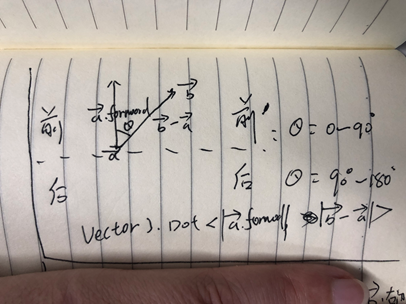Unity项目中的应用

1：通过点乘，我们可以计算出两个点之前的前后所属位置，当a·b>0;角度值在0到90度之间，可想而知及b点在a点的前方，反之a·b<0;角度值在90到180度之间，可想而知b点在a点的后方

2：可以根据点乘计算两个向量之间的夹角；<a,b>= arccos(a·b / (|a|·|b|))

话不多说看代码：

/// <summary>
/// 点乘
/// </summary>
/// <param name="a"></param>
/// <param name="b"></param>
private void TestDot(Transform a,Transform b)
{
//一个物体的坐标点也是有方向的  是和原点作为参考系
Vector3 vecAObj = a.transform.position;
Vector3 vecBObj = b.transform.position;

float dotResult = Vector3.Dot(vecAObj.normalized, vecBObj.normalized);
//将弧度转化为角度
Debug.Log("Vector3.Dot两个向量的角度为--------->" + angle);
Debug.Log("Vector3.Angle两个向量的角度为--------->" + Vector3.Angle(vecAObj, vecBObj));

Vector3 vecAPoint = a.transform.forward;
Vector3 vecBPoint = b.transform.position - a.transform.position;
float dotResult2 = Vector3.Dot(vecAPoint.normalized, vecBPoint.normalized);
if (dotResult2 > 0)
{
Debug.Log("b点在a点的前方-------->");
}
else {
Debug.Log("b点在a点的后方----------->");
}
Debug.Log("该两个点的角度值为---------->" + angle2);
}

2：叉乘：定义：c = a x b，其中a b c均为向量

几何意义是：得到一个与这两个向量都垂直的向量

性质1：c⊥a，c⊥b，即向量c与向量a，b所在平面垂直

性质2：模长|c| = |a||b| sin<a,b>

图片详解：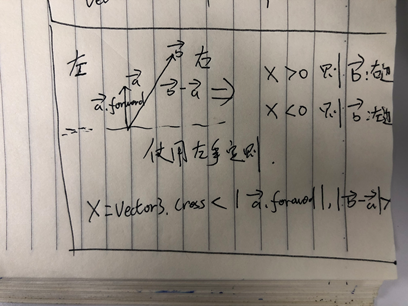Unity项目中的应用：

1.根据叉乘得到a，b向量的相对位置，和顺时针或逆时针方位。

简单的说: 点乘判断角度，叉乘判断方向。
形象的说: 当一个敌人在你身后的时候，叉乘可以判断你是往左转还是往右转更好的转向敌人，点乘得到你当前的面朝向的方向和你到敌人的方向的所成的角度大小。

/// <summary>
/// 叉乘
/// </summary>
private void TestCross(Transform a, Transform b)
{
Vector3 vecAobj = a.transform.forward;
Vector3 vecBObj = b.transform.position - a.transform.position;
Vector3 crossC = Vector3.Cross(vecAobj.normalized, vecBObj.normalized);
Debug.Log("该两个点的角度值为---------->" + angle);
if (crossC.y > 0)
{
Debug.Log("b在A的顺时针方向");// 由此可以用来判断我A点是向左转还是右转可以更快的找到B点。此处的顺时针和逆时针是指更快的速度找到对应点。 琢磨下 应该就清楚这里指什么意思了
}
else {
Debug.Log("b在A的逆时针方向");
}
}

展开全文• ## Unity点乘和叉乘的原理和使用

万次阅读 多人点赞 2016-12-13 15:14:43
Unity当中经常会用到向量的运算来计算目标的方位，朝向，角度等相关数据，下面咱们来通过实例学习下Unity当中最常用的点乘和叉乘的使用。 点乘 （又称"点积","数量积”,"内积"）（Dot Product, 用＊） 定义：a·b=...

Unity当中经常会用到向量的运算来计算目标的方位，朝向，角度等相关数据，下面咱们来通过实例学习下Unity当中最常用的点乘和叉乘的使用。

# 点乘

（又称"点积","数量积”,"内积"）（Dot Product, 用＊）

定义：a·b=|a|·|b|cos<a,b> 【注：粗体小写字母表示向量，<a,b>表示向量a,b的夹角，取值范围为[0，180]】

几何意义：是一条边向另一条边的投影乘以另一条边的长度.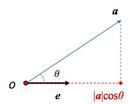v1v2向量的点乘运算：相应元素的乘积的和：v1( x1, y1，z1) * v2(x2, y2,z2) = x1*x2 + y1*y2+z1*z2;

注意 : 结果不是一个向量，而是一个标量。

性质1： a*b = |a||b|Cos(θ) θ是向量a 和向量 b之间的夹角。

性质2： a*b = b*a  满足乘法交换律

Unity项目应用：

1.根据点乘计算两个向量的夹角。<a,b>= arccos(a·b / (|a|·|b|))

2.根据点乘的正负值，得到夹角大小范围，>0，则夹角（0,90）<0,则夹角（90,180），可以利用这点判断一个多边形是面向摄像机还是背向摄像机。

3.根据点乘的大小，得到向量的投影长度，反应了向量的长度关系。

4.在生产生活中，点积同样应用广泛。利用点积可判断一个多边形是否面向摄像机还是背向摄像机。向量的点积与它们夹角的余弦成正比，因此在聚光灯的效果计算中，可以根据点积来得到光照效果，如果点积越大，说明夹角越小，则物理离光照的轴线越近，光照越强。物理中，点积可以用来计算合力和功。若b为单位矢量，则点积即为a在方向b的投影，即给出了力在这个方向上的分解。功即是力和位移的点积。计算机图形学常用来进行方向性判断，如两矢量点积大于0，则它们的方向朝向相近；如果小于0，则方向相反。矢量内积是人工智能领域中的神经网络技术的数学基础之一，此方法还被用于动画渲染（Animation-Rendering）。

# 叉乘

（又称"叉积","向量积","外积"）（cross product，用x）

定义：c = a x b，其中a b c均为向量

几何意义是：得到一个与这两个向量都垂直的向量，这个向量的模是以两个向量为边的平行四边形的面积

v1v2向量的叉乘运算：相应元素的乘积的和：v1( x1, y1，z1) x v2(x2, y2, z2) = (y1*z2 - y2*z1)i+(x2*z1 - x1*z2)j+(x1*y2-x2*y1)k;

利用三阶行列式计算

|i    j   k|

|x1 y1  z1|

|x2  y2  z2|

性质1：c⊥a，c⊥b，即向量c与向量a，b所在平面垂直

性质2：模长|c| = |a||b| sin<a,b>

性质3：(数学上)满足右手法则, a x b = -b x a，所以我们可以使用叉乘的正负值来判断a，b的相对位置，即b是处于a的顺时针还是逆时针方向。

叉乘的右手定则是用来确定叉乘积的方向的。

右手法则：右手的四指方向指向第一个矢量,屈向叉乘矢量的夹角方向（两个矢量夹角方向取小于180°的方向）,那么此时大拇指方向就是叉乘所得的叉乘矢量的方向.（大拇指应与食指成九十度）（注意：Unity当中使用左手，因为Unity使用的是左手坐标系）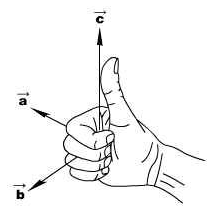数学上叉乘的右手法则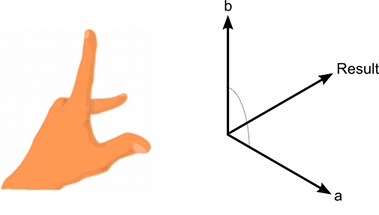Unity当中叉乘的左手法则

Unity项目应用：

1.根据叉乘得到a，b向量的相对位置，和顺时针或逆时针方位。

简单的说: 点乘判断角度，叉乘判断方向。
形象的说: 当一个敌人在你身后的时候，叉乘可以判断你是往左转还是往右转更好的转向敌人，点乘得到你当前的面朝向的方向和你到敌人的方向的所成的角度大小。

2.得到a，b夹角的正弦值，计算向量的夹角（0,90），可以配合点乘和Angle方法计算出含正负的方向。

3.根据叉乘大小，得到a，b向量所形成的平行四边形的面积大小，根据面积大小得到向量的相对大小。

# 下面是代码

using UnityEngine;

public class VectorExample : MonoBehaviour {

//点积
private void TestDot(Vector3 a, Vector3 b)
{
// 计算 a、b 点积结果
float result = Vector3.Dot(a, b);

// 通过向量直接获取两个向量的夹角（默认为 角度）， 此方法范围 [0 - 180]
float angle = Vector3.Angle(a, b);

// 计算 a、b 单位向量的点积,得到夹角余弦值,|a.normalized|*|b.normalized|=1;
result = Vector3.Dot(a.normalized, b.normalized);
// 通过反余弦函数获取 向量 a、b 夹角（默认为 弧度）
// 将弧度转换为 角度
}

//叉乘
private void TestCross(Vector3 a, Vector3 b)
{
//计算向量 a、b 的叉积，结果为 向量
Vector3 c = Vector3.Cross(a, b);

// 通过反正弦函数获取向量 a、b 夹角（默认为弧度）
float radians = Mathf.Asin(Vector3.Distance(Vector3.zero, Vector3.Cross(a.normalized, b.normalized)));

// 判断顺时针、逆时针方向，是在 2D 平面内的，所以需指定一个平面，
//下面以X、Z轴组成的平面为例 , (Y 轴为纵轴),
// 在 X、Z 轴平面上，判断 b 在 a 的顺时针或者逆时针方向,
if (c.y > 0)
{
// b 在 a 的顺时针方向
}
else if (c.y == 0)
{
// b 和 a 方向相同（平行）
}
else
{
// b 在 a 的逆时针方向
}
}

// 获取两个向量的夹角  Vector3.Angle 只能返回 [0, 180] 的值
// 如真实情况下向量 a 到 b 的夹角（80 度）则 b 到 a 的夹角是（-80）
// 通过 Dot、Cross 结合获取到 a 到 b， b 到 a 的不同夹角
private void GetAngle(Vector3 a, Vector3 b)
{
Vector3 c = Vector3.Cross(a, b);
float angle = Vector3.Angle(a, b);

// b 到 a 的夹角
float sign = Mathf.Sign(Vector3.Dot(c.normalized, Vector3.Cross(a.normalized, b.normalized)));
float signed_angle = angle * sign;

Debug.Log("b -> a :" + signed_angle);

// a 到 b 的夹角
sign = Mathf.Sign(Vector3.Dot(c.normalized, Vector3.Cross(b.normalized, a.normalized)));
signed_angle = angle * sign;

Debug.Log("a -> b :" + signed_angle);
}
}


展开全文• 本文从http://blog.csdn.net/yupu56/article/details/53609028转载而来Unity当中经常会用到向量的运算来计算目标的方位，朝向，角度等相关数据，下面咱们来通过实例学习下Unity当中最常用的点乘和叉乘的使用。...
• Unity中点乘和叉乘点乘（API: Vector3.Dot()）点乘的计算公式点乘的几何意义用途之一：判断一个物体当前方位利用点乘求出角度叉乘（API: Vector3.Cross()）叉乘计算公式叉乘的几何意义判断物体是在左侧还是右侧 点乘...
• Unity中的点乘和叉乘 点乘很多资料上都是说表示两个向量的相似度，具体是怎么表示相似度，结果大小如何表示相似度，越大越相似，还是越差，如果细细去推导才发现很多东西以前的理解是有错误或者说是不清不楚的。 ...
•游戏开发
• 比如敌人再附近，点乘可以求出玩家面朝方向敌人方向的夹角，叉乘可以得出左转还是右转更好的转向敌人 Part 1 点乘： 数学上的 点乘为 a * b = |a| * |b| * cos(Θ) Unity中的点乘也是如此 点乘结果为 ...游戏开发 笔记
• Unity游戏开发——向量运算（点乘和叉乘） 0.前言 在游戏开发中，确定目标得方向，朝向，夹角等相关数据需要用到向量的计算，其中最常用到的就时点乘和叉乘。 因为我们主要研究的是游戏开发中的3D世界向量，所以小面...游戏开发
• ## 向量点乘和叉乘

千次阅读 2021-05-02 10:56:12
点乘和叉乘unity中有广泛的应用：结论点乘判断角度，叉乘判断朝向方位。点乘：结果为一个常数又称"点积","数量积”,“内积”(Dot Product, 用＊)对于向量 A = (x1, y1, z1) ，向量 B = (x2, y2, z2),则向量A点乘...
• 当向量a向量b归一化后，点乘的值：同向为1（夹角为0），垂直为0（夹角为90），负向为-1（夹角为180）。 点乘用法： 1、得到2个向量的夹角：范围[0, 180] ，可以做游戏怪物的视角是否有查看到玩家，可以用来计算...
• 在游戏开发中，确定目标的方位，朝向，夹角等相关数据需要用到向量间的计算，其中最常用到的就是点乘和叉乘。 说实话，因为向量的运算在平时开发中不是经常使用，我也总弄混这两种运算的含义，所以这次好好区分一下...游戏引擎
• 1、点乘 定义：a*b=|a||b|cos<a,b> 几何意义：一条边向另一条边的投影乘以另一条边的长度 运算：x1*x2+y1*y2+z1*z2....1、根据点乘计算两个向量的...2、叉乘 定义：axb=c，三个都是向量 几何意义：结果是一个.
• 点乘：两 个向量点乘得到一个标量 ,数 值等于两个 。 b  向量长度桕乘后再乘以二者夹角的佘弦值 。如果两 个 向量a,b均 为单位 向量 ,那 么a.b等 于向量b在 向 量a方 向上的投影的长度
• Unity3D 点乘 ：Vector3.Dot，叉乘：Vector3.Cross 假设有向量a，b cos，b>表示a，b组成的余玄值 |a|表示向量a的长度 点乘a.b = |a||b|cos，b>。如果a，b都是单位向量，那么点乘表示a在b上投影的长度。 ...
• 两个向量的点乘，就是我们说的数量级 a·b=|a|·|b|cosθ 结果是一个标量，如果==0，则两个向量夹角等于90度，垂直关系 小于0，则两个向量夹角大于90度 大于0，则两个向量夹角小于90度 如果cosθ等于-1，则两个...
• 点乘： 意义：可以判断目标物体是否在我的前方;    Vector3.Dot(transform.forward, Target.position-transform.position)大于零表示在我前面，小于零表示在我后面 等于零表示在我正左边或者正右边。   ...
• 点乘：两个向量点乘得到一个标量 ，数值等于两个向量长度相乘后再乘以二者夹角的余弦值 。如果两个向量a,b均 为单位 向量 ,那么a.b等于向量b在向量a方向...叉乘：两 个向量的叉乘得到一个新的向量 ,新向量垂直于原来的
• 点乘的应用： （1） 点乘可以用来判断两个向量是否垂直，返回值为0，则垂直。（公式：abcosθ，向量夹角θ为90度，则垂直） （2）本质上其实是判断两个向量相似的程度（2个向量夹角越小，越相似）。 （3）计算...
• 然后再根据自己forward这个向量计算自己与敌人之是不是并头 float f2 = Vector3.Angle(this.transform.forward, b2 - b1);//得到两者间的角度 if (f > 0 && b3.y > 0) { Debug.Log("红球在自己右前" + f2 + "度");...
• 今天豁然开朗来记录一下我理解的矩阵以及四元数和点乘叉乘的理解。 首先说矩阵以及四元数 矩阵的乘法是根据n*m列来获取的，n的行数是代表结果的行数，m的列数代表结果的列数。 红色与黑色表示第一个矩阵中...
• 先阐述一下向量的概念公式，再结合例子去验证这些公式1、向量的模：向量的大小就是向量各分量平方的平方根。向量的模公式： 设 a = (x, y, z),|a|= sqr(x * x + y * y + z * z);对应unity中的Vector3.magnitude...
• Unity当中经常会用到向量的运算来计算目标的方位，朝向，角度等相关数据，下面咱们来通过实例学习下Unity当中最常用的点乘和叉乘的使用。 点乘 （又称”点积”,”数量积”,”内积”）（Dot Product, 用＊） 定义...
• 向量的点乘和叉乘 Unity3D 点乘 ：Vector3.Dot，叉乘：Vector3.Cross 名称 标积 / 内积 / 数量积 / 点乘 矢积 / 外积 / 向量积 / 叉乘 运算式（a，bc粗体字，表示向量） a·b=|a||b|·cosθ a×b=c,其中|c|=|a||b...坐标系 向量
• Unity中经常会用到向量的运算来计算目标的方位，朝向，角度等相关数据，而这些计算中最常用的就是点乘和叉乘a·b=|a|·|b|cos 【注：小写字母表示向量，表示向量a,b的夹角，取值范围为[0，180]】一条边向另一条边的...
• 点乘叉乘数学原理性的东西比较“难记”，网上很多。实用举例，网上算是比较少吧。故，来总结一番。 2.可以解决的问题 　I.如何计算角度 　II.如何判断前后 　III.如何判断逆时针还是顺时针。 　IV.如何判断其他......